Examples

Chapter 4 Class 10 Quadratic Equations
Serial order wise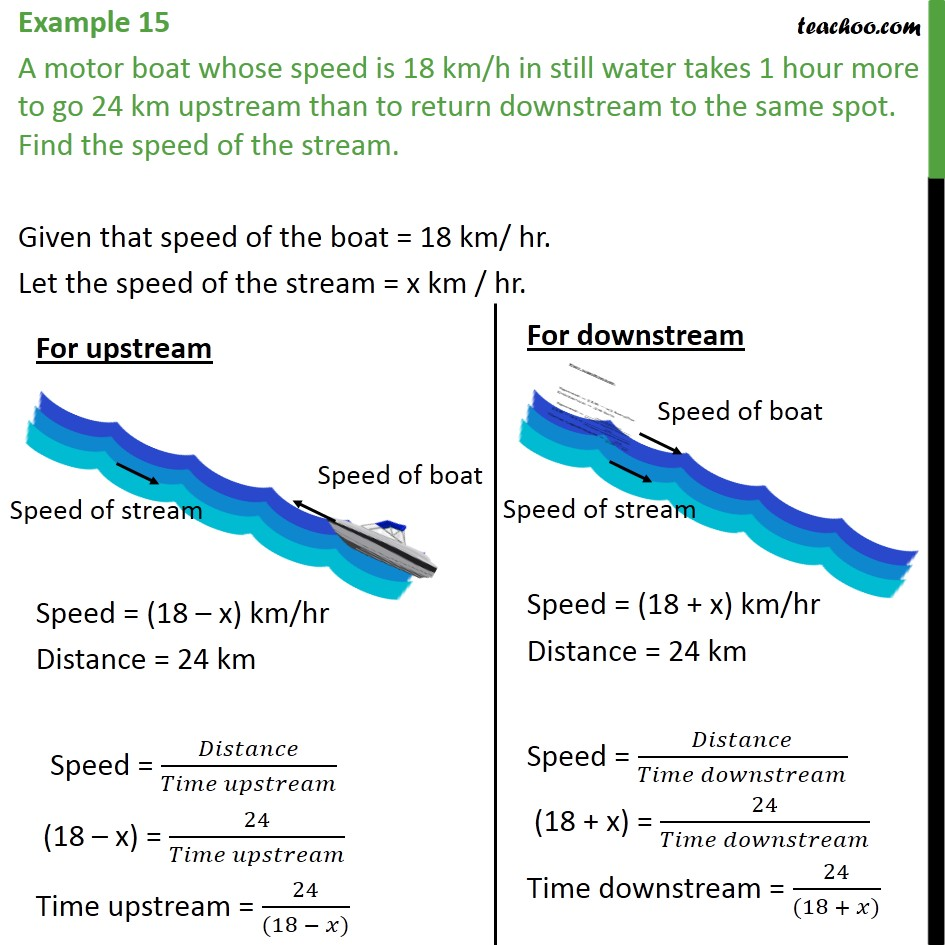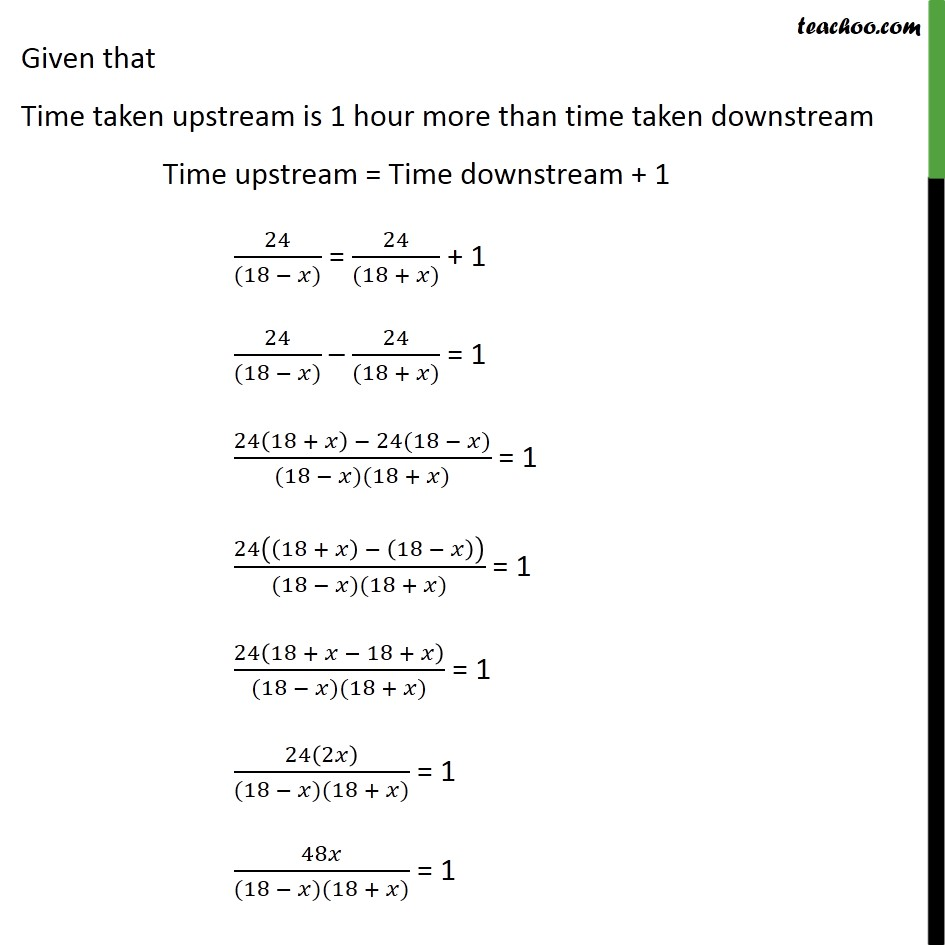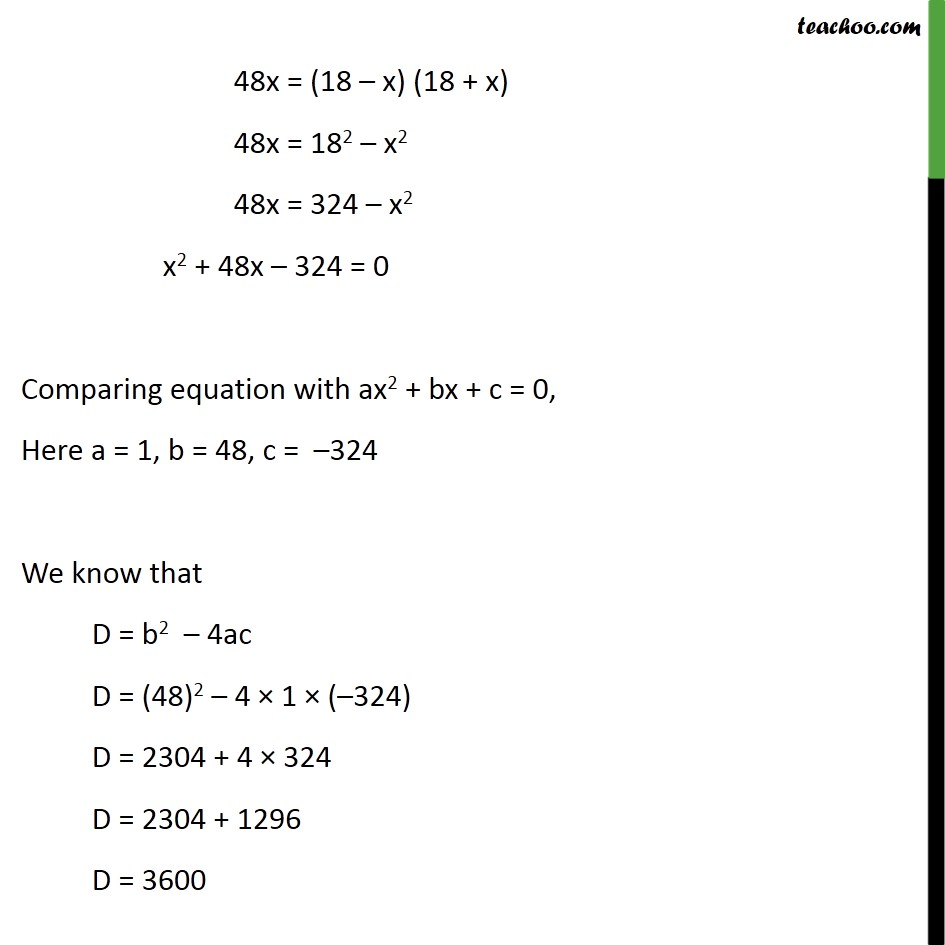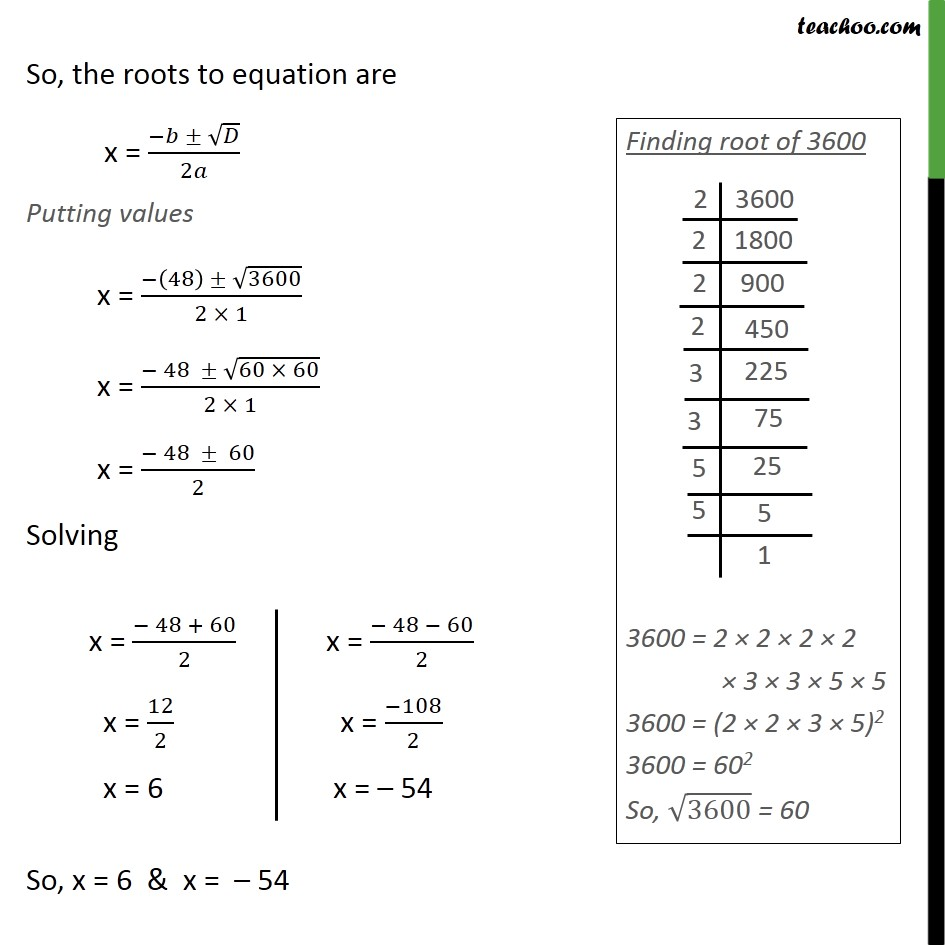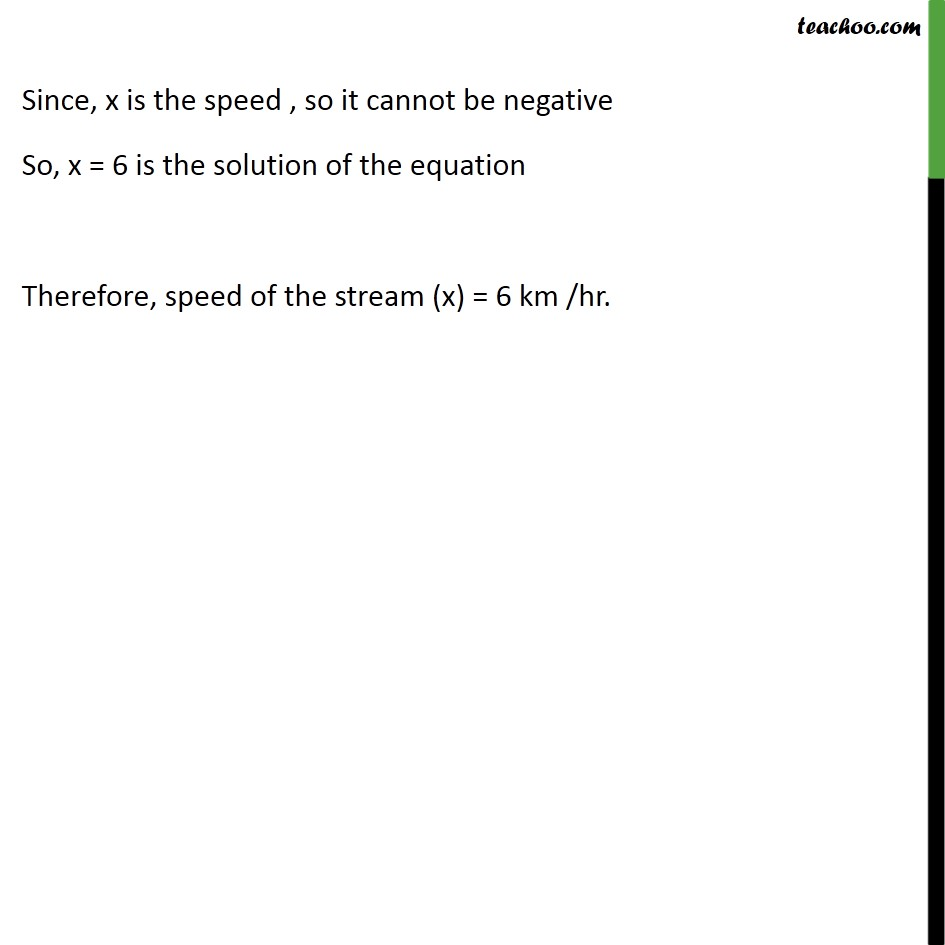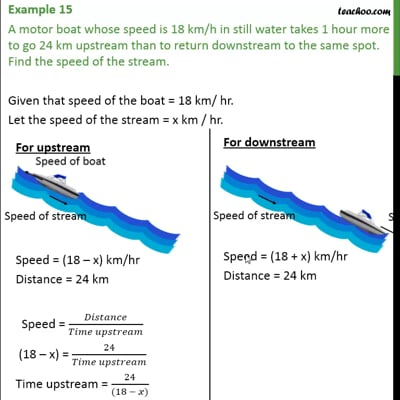This video is only available for Teachoo black users

Learn in your speed, with individual attention - Teachoo Maths 1-on-1 Class

### Transcript

Question 8 A motor boat whose speed is 18 km/h in still water takes 1 hour more to go 24 km upstream than to return downstream to the same spot. Find the speed of the stream. Given that speed of the boat = 18 km/ hr. Let the speed of the stream = x km / hr. Given that Time taken upstream is 1 hour more than time taken downstream Time upstream = Time downstream + 1 24/((18 − 𝑥)) = 24/((18 + 𝑥)) + 1 24/((18 − 𝑥)) – 24/((18 + 𝑥)) = 1 (24(18 + 𝑥) − 24(18 − 𝑥))/((18 − 𝑥)(18 + 𝑥)) = 1 24((18 + 𝑥) − (18 − 𝑥))/((18 − 𝑥)(18 + 𝑥)) = 1 24(18 + 𝑥 − 18 + 𝑥)/((18 − 𝑥)(18 + 𝑥)) = 1 24(2𝑥)/((18 − 𝑥)(18 + 𝑥)) = 1 48𝑥/((18 − 𝑥)(18 + 𝑥)) = 1 48x = (18 – x) (18 + x) 48x = 182 – x2 48x = 324 – x2 x2 + 48x – 324 = 0 Comparing equation with ax2 + bx + c = 0, Here a = 1, b = 48, c = –324 We know that D = b2 – 4ac D = (48)2 – 4 × 1 × (–324) D = 2304 + 4 × 324 D = 2304 + 1296 D = 3600 So, the roots to equation are x = (−𝑏 ± √𝐷)/2𝑎 Putting values x = (−(48) ± √3600)/(2 × 1) x = (− 48 ± √(60 × 60))/(2 × 1) x = (− 48 ± 60)/2 Solving So, x = 6 & x = – 54 Since, x is the speed , so it cannot be negative So, x = 6 is the solution of the equation Therefore, speed of the stream (x) = 6 km /hr.